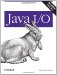# Parsing Input

Number formats also handle input. When used for input, a number format converts a string in the appropriate format to a binary number, achieving more flexible conversions than you can get with the methods in the type wrapper classes (like Integer.parseInt( )). For instance, a percent format parse( ) method can interpret 57% as 0.57 instead of 57. A currency format can read (12.45) as -12.45.

There are three parse( ) methods in the NumberFormat class. All do roughly the same thing:

```public Number parse(String text) throws ParseException
public abstract Number parse(String text, ParsePosition parsePosition)
public final Object parseObject(String source, ParsePosition parsePosition)
```

The first parse( ) method attempts to parse a number from the given text. If the text represents an integer, its returned as an instance of java.lang.Long. Otherwise, its returned as an instance of java.lang.Double. If a string contains multiple numbers, only the first one is returned. For instance, if you parse "32 meters" youll get the number 32 back. Java throws away everything after the number finishes. If the text cannot be interpreted as a number in the given format, a ParseException is thrown.

The second parse( ) method specifies where in the text parsing starts. The position is given by a ParsePosition object. This is a little more complicated than using a simple int but does have the advantage of allowing one to read successive numbers from the same string. The third parse( ) method merely invokes the second. Its declared to return Object rather than Number so that it can override the method of the same signature in java.text.Format. If you know you e working with a NumberFormat rather than a DateFormat or some other nonnumeric format, theres no reason to use it.

The java.text.ParsePosition class has one constructor and two public methods:

```public ParsePosition(int index)
public int getIndex( )
public void setIndex(int index)
```

This whole class is just a wrapper around a position, which is set by the constructor and the setIndex( ) method and returned by the getIndex( ) method. As a NumberFormat parses a string, it updates the associated ParsePositions index. Thus, when passed into a parse( ) method, the ParsePosition contains the index where parsing will begin. When the parse( ) method returns, the ParsePosition contains the index immediately after the last character parsed. If parsing fails, the parse position is unchanged.

Some number formats can only read integers, not floating-point numbers. The isParseIntegerOnly( ) method returns TRue if this is the case or false otherwise.

```public boolean isParseIntegerOnly( )
public void setParseIntegerOnly(boolean value)
```

The setParseInteger( ) method lets you specify that the format should only parse integers. If a decimal point is encountered, parsing should stop.

Example 21-8 is a simple program of the sort thats common in CS 101 courses. The assignment is to write a program that reads a number entered from the command line and prints its square root. Successive numbers are read until a negative number is entered, at which point the program halts. Although this is a very basic exercise, its relatively complex in Java because Java separates string parsing from basic I/O. Nonetheless, while it may not be suitable for the first weeks homework, students should be able to handle it by the end of the semester.

##### Example 21-8. RootFinder

 ```import java.text.*; import java.io.*; public class RootFinder { public static void main(String[] args) { Number input = null; try { BufferedReader br = new BufferedReader(new InputStreamReader(System.in)); NumberFormat nf = NumberFormat.getInstance( ); while (true) { System.out.println("Enter a number (-1 to quit): "); String s = br.readLine( ); try { input = nf.parse(s); } catch (ParseException ex) { System.out.println(s + " is not a number I understand."); continue; } double d = input.doubleValue( ); if (d < 0) break; double root = Math.sqrt(d); System.out.println("The square root of " + s + " is " + root); } } catch (IOException ex) {System.err.println(ex);} } } ```

Heres a sample run:

```\$ java RootFinder
Enter a number (-1 to quit):
87
The square root of 87 is 9.327379053088816
Enter a number (-1 to quit):
3.151592
The square root of 3.151592 is 1.7752723734683644
Enter a number (-1 to quit):
2,345,678
The square root of 2,345,678 is 1531.5606419596973
Enter a number (-1 to quit):
2.998E+8
The square root of 2.998E+8 is 1.7314733610425546
Enter a number (-1 to quit):
299800000
The square root of 299800000 is 17314.733610425545
Enter a number (-1 to quit):
0.0
The square root of 0.0 is 0.0
Enter a number (-1 to quit):
four
four is not a number I understand.
Enter a number (-1 to quit):
4
The square root of 4 is 2.0
Enter a number (-1 to quit):
(12)
(12) is not a number I understand.
Enter a number (-1 to quit):
-1
```

These results tell you a few things about Javas default number format in the locale where I ran it (U.S. English). First, it doesn understand exponential notation. The square root of 2.998E+8 is not 1.7314733610425546; its 1.7314733610425546E+4. The number format parsed up to the first character it didn recognize (E) and stopped, thus returning the square root of 2.998 instead. You can also see that this number format doesn understand negative numbers represented by parentheses or words like "four." On the other hand, it can parse numbers with thousands separators like 2,345,678. This is more than the I/O libraries in most other languages can do. With the appropriate, nondefault number format, Java could parse (12), four, and 2.998E+8 as well.Java I/O ISBN: 0596527500EAN: 2147483647 Year: 2004Pages: 244 Authors: Elliotte Rusty Harold BUY ON AMAZON Flylib.com © 2008-2020. If you may any questions please contact us: flylib@qtcs.net